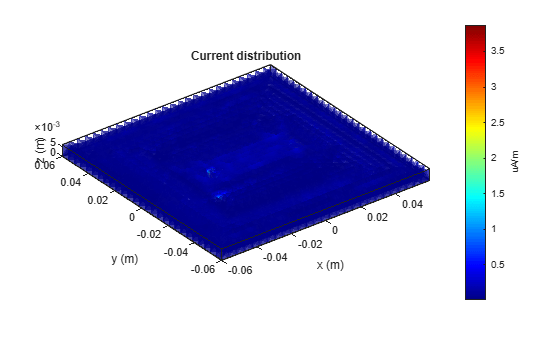# current

Current distribution on metal or dielectric antenna or array surface

## Syntax

``current(object,frequency)``
``i = current(object,frequency)``
``[i,p] = charge(object,frequency)``
``current(object,frequency,'dielectric')``
``i = current(object,frequency,'dielectric')``
``i = current(___,Name,Value)``

## Description

example

````current(object,frequency)` calculates and plots the absolute value of the current on the surface of an antenna or array object, at a specified frequency.```

example

````i = current(object,frequency)` returns the x, y, z components of the current on the surface of an antenna or array object, at a specified frequency.```
````[i,p] = charge(object,frequency)` returns the x, y, z components of the current on the surface of an antenna or array object, at a specified frequency and at the point in which the current calculation is performed.```

example

````current(object,frequency,'dielectric')` calculates and plots the absolute value of current at a specified frequency value on the dielectric face of the antenna or array.```
````i = current(object,frequency,'dielectric')` returns the x, y, z components of the current on the dielectric surface of an antenna or array object, at a specified frequency.```
````i = current(___,Name,Value)` calculates the current on the surface of an antenna using additional name-value pairs.```

## Examples

collapse all

Calculate and plot the current distribution for a circular loop antenna at 70MHz frequency.

``` h = loopCircular; current(h,70e6);```Calculate the current distribution of a default rectangular array at 70MHz frequency.

```h = rectangularArray; i = current(h,70e6)```
```i = 3×160 complex 0.0000 + 0.0000i 0.0000 + 0.0000i 0.0000 + 0.0000i 0.0000 + 0.0000i 0.0000 + 0.0000i 0.0000 + 0.0000i 0.0000 + 0.0000i 0.0000 + 0.0000i 0.0000 + 0.0000i 0.0000 + 0.0000i 0.0000 + 0.0000i 0.0000 + 0.0000i 0.0000 + 0.0000i 0.0000 + 0.0000i 0.0000 + 0.0000i 0.0000 + 0.0000i 0.0000 + 0.0000i 0.0000 + 0.0000i 0.0000 + 0.0000i 0.0000 + 0.0000i 0.0000 + 0.0000i 0.0000 + 0.0000i 0.0000 + 0.0000i 0.0000 + 0.0000i 0.0000 + 0.0000i 0.0000 + 0.0000i 0.0000 + 0.0000i 0.0000 + 0.0000i 0.0000 + 0.0000i 0.0000 + 0.0000i 0.0000 + 0.0000i 0.0000 + 0.0000i 0.0000 + 0.0000i 0.0000 + 0.0000i 0.0000 + 0.0000i 0.0000 + 0.0000i 0.0000 + 0.0000i 0.0000 + 0.0000i 0.0000 + 0.0000i 0.0000 + 0.0000i 0.0000 + 0.0000i 0.0000 + 0.0000i 0.0000 + 0.0000i 0.0000 + 0.0000i 0.0000 + 0.0000i 0.0000 + 0.0000i 0.0000 + 0.0000i 0.0000 + 0.0000i 0.0000 + 0.0000i 0.0000 + 0.0000i 0.0009 + 0.0020i 0.0013 + 0.0025i -0.0002 - 0.0012i 0.0003 + 0.0013i 0.0004 + 0.0015i -0.0005 - 0.0015i -0.0014 - 0.0025i -0.0009 - 0.0020i 0.0015 + 0.0027i -0.0015 - 0.0027i 0.0030 + 0.0051i -0.0020 - 0.0034i 0.0017 + 0.0030i -0.0017 - 0.0030i -0.0000 - 0.0041i 0.0000 + 0.0021i -0.0007 - 0.0017i 0.0007 + 0.0017i 0.0011 + 0.0023i -0.0011 - 0.0023i 0.0007 + 0.0017i -0.0007 - 0.0018i 0.0005 + 0.0015i -0.0005 - 0.0015i 0.0011 + 0.0022i 0.0003 + 0.0013i -0.0009 - 0.0020i 0.0009 + 0.0020i -0.0015 - 0.0027i 0.0016 + 0.0028i -0.0002 - 0.0012i -0.0011 - 0.0023i -0.0016 - 0.0030i 0.0017 + 0.0029i 0.0001 + 0.0021i -0.0000 - 0.0041i 0.0030 + 0.0051i -0.0013 - 0.0025i -0.0020 - 0.0035i 0.0013 + 0.0025i 0.0009 + 0.0020i 0.0013 + 0.0025i -0.0002 - 0.0012i 0.0003 + 0.0013i 0.0005 + 0.0015i -0.0005 - 0.0015i -0.0013 - 0.0025i -0.0009 - 0.0020i 0.0015 + 0.0027i -0.0015 - 0.0027i 0.0562 + 0.1041i 0.0428 + 0.0763i 0.0659 + 0.1334i 0.0649 + 0.1280i 0.0641 + 0.1250i 0.0621 + 0.1188i 0.0373 + 0.0658i 0.0523 + 0.0956i 0.0343 + 0.0603i 0.0280 + 0.0487i 0.0031 + 0.0054i 0.0137 + 0.0235i 0.0176 + 0.0302i 0.0246 + 0.0427i 0.0662 + 0.1423i 0.0661 + 0.1358i 0.0580 + 0.1081i 0.0609 + 0.1154i 0.0453 + 0.0813i 0.0501 + 0.0911i 0.0608 + 0.1154i 0.0579 + 0.1079i 0.0621 + 0.1188i 0.0641 + 0.1251i 0.0502 + 0.0910i 0.0649 + 0.1281i 0.0523 + 0.0955i 0.0562 + 0.1040i 0.0344 + 0.0603i 0.0280 + 0.0487i 0.0659 + 0.1334i 0.0455 + 0.0816i 0.0247 + 0.0427i 0.0177 + 0.0303i 0.0661 + 0.1358i 0.0662 + 0.1422i 0.0032 + 0.0054i 0.0373 + 0.0658i 0.0138 + 0.0236i 0.0429 + 0.0765i 0.0562 + 0.1041i 0.0428 + 0.0763i 0.0659 + 0.1334i 0.0649 + 0.1280i 0.0641 + 0.1250i 0.0621 + 0.1188i 0.0373 + 0.0658i 0.0523 + 0.0956i 0.0343 + 0.0602i 0.0280 + 0.0487i ```

Create a microstrip patch antenna using 'FR4' as the dielectric substrate.

```d = dielectric('FR4'); pm = patchMicrostrip('Length',75e-3, 'Width',37e-3, ... 'GroundPlaneLength',120e-3, 'GroundPlaneWidth',120e-3, ... 'Substrate',d)```
```pm = patchMicrostrip with properties: Length: 0.0750 Width: 0.0370 Height: 0.0060 Substrate: [1x1 dielectric] GroundPlaneLength: 0.1200 GroundPlaneWidth: 0.1200 PatchCenterOffset: [0 0] FeedOffset: [-0.0187 0] Tilt: 0 TiltAxis: [1 0 0] Load: [1x1 lumpedElement] ```
`show(pm)`Plot the current distribution on the antenna at a frequency of 1.67 GHz.

```figure current(pm,1.67e9,'dielectric')```Create a default pifa (planar inverted F antenna).

`ant = pifa;`

Visualize the current distribution on the pifa antenna in using log function scale.

`current(ant,1.75e9,'scale','log')`## Input Arguments

collapse all

Antenna or array object, specified as a scalar handle.

Frequency to calculate current distribution, specified as a scalar in Hz.

Example: 70e6

Data Types: `double`

### Name-Value Pair Arguments

Specify optional comma-separated pairs of `Name,Value` arguments. `Name` is the argument name and `Value` is the corresponding value. `Name` must appear inside quotes. You can specify several name and value pair arguments in any order as `Name1,Value1,...,NameN,ValueN`.

Example: `'scale','log10'`

Scale to visualize the current distribution on the surface of the antenna, specified as a string or a function handle. The string values are: `'linear'`, `'log'`, `'log10'`. By default, the value is `'linear'`. The function handle can be of any mathematical function such as `log`, `log10`, `cos`, or `sin`.

Data Types: `char` | `function_handle`

## Output Arguments

collapse all

x, y, z components of current distribution, returned as a 3-by-n complex matrix in A/m. The value of the current is calculated on every triangle mesh or every dielectric tetrahedron face on the surface of an antenna or array.

Cartesian coordinates representing the center of each triangle in the mesh, returned as a 3-by-n real matrix.